# How to Find the Area of Rectangles

1. Math Lessons >
2. Area of Rectangles

### Why Find the Area of Rectangles?

Before we dive into the lesson, let’s brush up a little on why we find the area of rectangles and where we use it. This is Joe’s kitchen garden.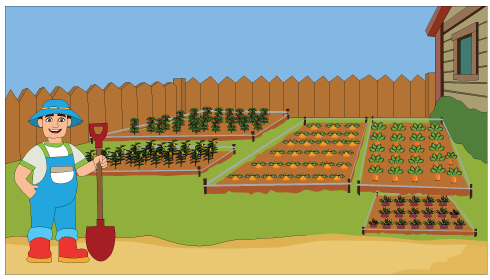Let’s zoom in on the beet patch and the carrot patch.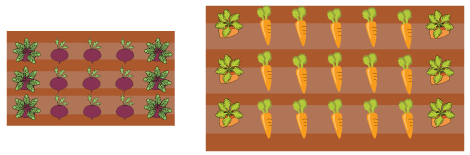Can you tell which is bigger? You’re right. It’s the carrot patch! You said that with just a glimpse of them, didn’t you?
Now, take a look at these two patches of veggies.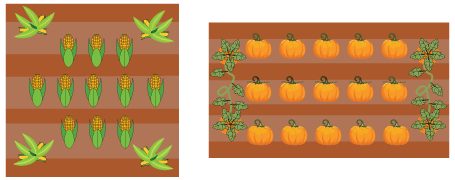Can you identify which of them is bigger? That’s a bit tricky, isn’t it?
In this case, we can’t tell which is bigger by just looking at them. We need to calculate the area.

The area of a rectangle is the amount of space the rectangle or its surface occupies.

### How to Find the Area of Rectangles by Counting Squares

A simpler way of finding the area of rectangle is using a grid. Let’s take a look at how Joe calculates the area of his pumpkin patch using a grid.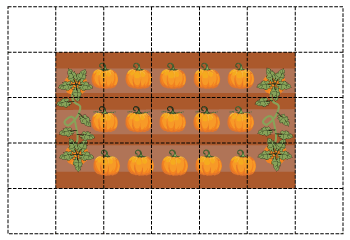The boxes you see on this grid are called unit squares. A unit square is a square whose side length is 1 unit. To determine the area, we need to figure out the total number of unit squares occupied by this rectangular patch.

In the above grid, the rectangle is composed of 15 unit squares. Hence, the area of the patch is 15 square units.

But counting squares to determine the area is not always feasible. We need to look for a simpler method.

### How to Find the Area of Rectangles Using Formula

Take a look at the grid once again.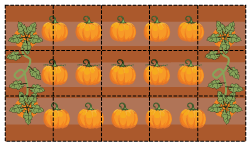There are 5 columns and 3 rows: 5 unit squares along the length and 3 unit squares along the width. Now the total number of unit squares that the pumpkin patch occupies is:

5 × 3 = 15 unit squares

We’ll assume that the length of each unit square is 1 yard, so their area is 1 square yard. Then Joe’s pumpkin patch will have:

A length of 5 yards

A width of 3 yards

An area of 15 square yards (5 yards × 3 yards)

Hang on! What did we do now?

You’re right! We’ve derived a formula to find the area of a rectangle.

Area of the rectangle = length × width

A = l × w

All we need to do is just plug the dimensions and figure out the area. And yes! This works much faster than counting squares.

Now let’s take a look at a few examples that have been solved using the formula.

Example 1

Find the area of Joe’s rectangular bell pepper patch.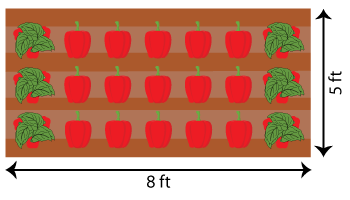The length of the bell pepper patch is 8 feet and its width is 5 feet.

We know that, Area of the rectangle = length × width.

Plugging in the length and width we have,

A = 8 ft × 5 ft

A = 40 ft2

Thus, the area of Joe’s bell pepper patch is 40 square feet.

Example 2

Determine the area of Joe’s square-shaped corn patch.Each side of the square patch measures 9 feet.

The length and width in the case of a square are the same.

So our formula becomes, Area of the square = side length × side length or A = s2.

A = 9 ft × 9 ft

A = 81 ft2

Thus, the area of Joe’s square corn patch is 81 square feet.

Example 3

Determine the area of this rectangular figure.The dimensions of the rectangle are l = 11 yd and w = 14 yd.

We know that, A = l × w.

A = 11 yd × 14 yd   [Substituting l and w]

A = 154 yd2

Therefore, the area of this rectangle is 154 square yards.

### How to Find the Missing Length or Width of a Rectangle Using the Area

Just as we calculated the area of a rectangle using the length and width, we can find either the length or width of a rectangle using the area, provided that one of the dimensions is known. To find the width, divide the area by length; to find the length, divide the area by width.

Let’s take a look at this scenario.

As part of an everyday review, Sam buys a rectangular map poster highlighting major rivers of the world. The poster occupies an area of 864 square inches. If it has a width of 24 inches, what is its height?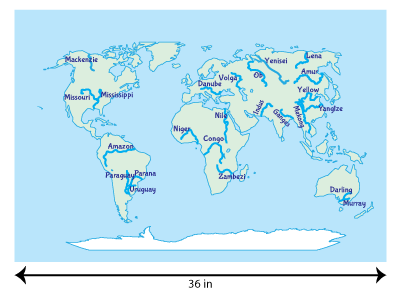Here, the width of the map is 24 inches, and its area is 864 square inches.

Now, the area of our map would be width times height, A = w × h.

But, we need the height of the map. So, let's rearrange the formula with h as the subject.

h = Aw

h = 864 in224 in

h = 36 in

Thus, the height of the map poster is 36 inches.

1) A standard basketball court is 94 feet long and 50 feet wide. How much is its area?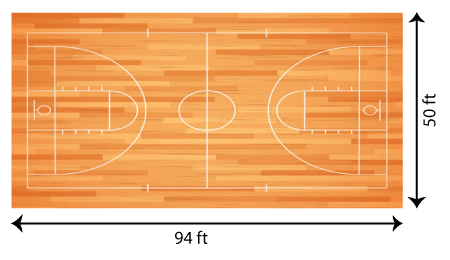The length of the court is 94 ft, and the width is 50 ft.

Let's recall our formula, Area of the rectangle = length × width.

Substituting the length and width of the court, we get:

A = 94 ft × 50 ft

A = 4,700 ft2

The area of the court is 4,700 square feet.

2) Jeremiah tiles the rectangular floor of her living room using 1-foot tiles as shown. The floor’s width is 11 feet, and its area is 132 square feet. What is the length?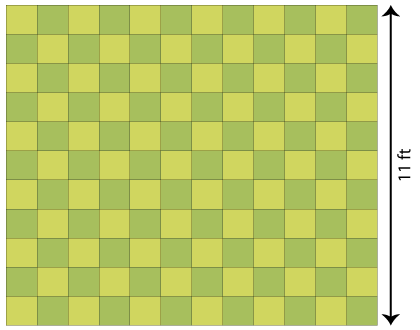The area of the rectangular floor (A) is 132 square feet, and its width (w) is 11 ft.

Now, the length of the floor (l) can be determined using the formula, l = Aw.

Substituting the measures, we get:

l = 132 ft211 ft

l = 12 ft

Thus, the length of the floor is 12 feet.

### A Brief Look at the Lesson

• The area of a rectangle is the amount of space the rectangle or its surface occupies.

• We can calculate the area of a rectangle by counting squares on the grid or by multiplying the length and width.

• To find the length of the rectangle, divide the area by width; to find the width, divide the area by length.

• A rectangle that has the same length and width is a square.Crank up your skills with our free printable Area of Rectangles worksheets.

Progress
Score

Restart Quiz